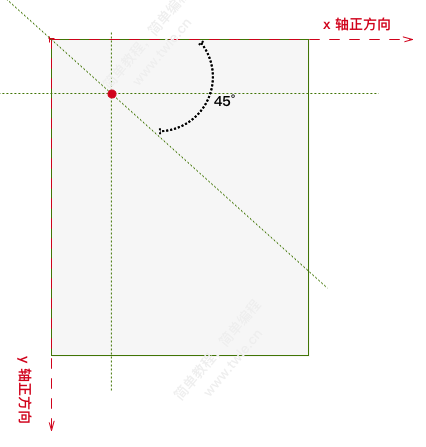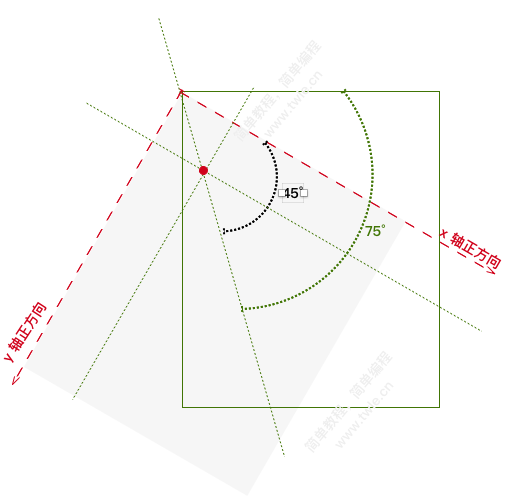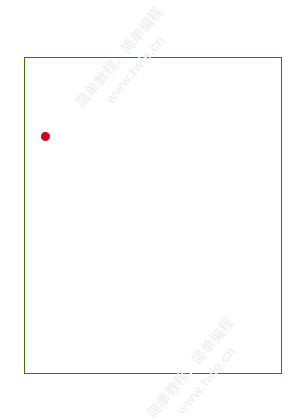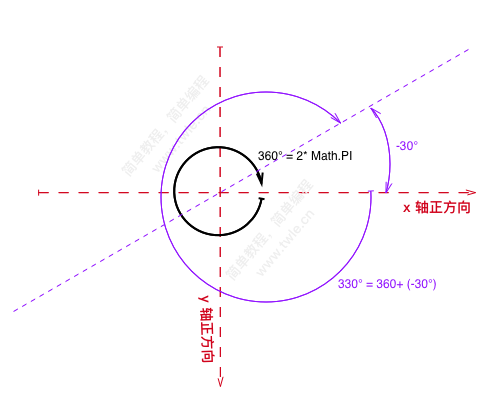Canvas 旋转 rotate()Canvas rotate()

`ctx.rotate()``canvas` 围绕屏幕左上角(0,0) 顺时针旋转一定角度

语法

```void ctx.rotate(angle);
```

angle 顺时针旋转的弧度。可以通过角度值计算：`degree * Math.PI / 180`

弧度 angle

```angle = 30 * Math.PI / 180
```

```angle = 360 + ( -30 * Math.PI / 180 )
``````3530° % 360 = 290°
```

```3530° / 360 = 9.8 = 9
```

范例

```<!DOCTYPE html>
<meta charset="utf-8">
<canvas id="canvas-1" width="400" height="300">
</canvas>

<script>
var c   = document.getElementById("canvas-1");
var ctx = c.getContext("2d");
ctx.fillStyle="orange";
ctx.fillRect(50,50,100,50);
ctx.rotate(30 * Math.PI / 180);
ctx.fillStyle="green";
ctx.fillRect(50,50,100,50);
</script>
```

范例 2

```<!DOCTYPE html>
<meta charset="utf-8">
<canvas id="canvas-2" width="400" height="00">
</canvas>

<script>
var c   = document.getElementById("canvas-2");
var ctx = c.getContext("2d");
var colors = ['#D0021B','#F5A623','#8B572A','#417505','#9013FE','#000000']
for ( var i = 0; i < colors.length; i++ )
{
ctx.fillStyle = colors[i];
ctx.fillRect(0,0,200,50);
ctx.rotate(15 * Math.PI / 180);
}
</script>
```

Canvas 基础教程

简单教程，简单编程 - IT 入门首选站